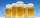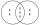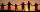# Divisibility + multiplication - math problems

#### Number of problems found: 34

• Four multiplesFour multiples of 6, he writes the following 12  24  56  72, which is correct?
• Three-digit numbersHow many are all three-digit numbers that are made up of digits 0,2,5,7 and are divisible by 9 if the digits can be repeated?
• We rollWe roll two dice A. - what is the probability that the sum of the falling numbers is at most 4 B. - is at least 10 C. - is divisible by 5?
• Even/odd numbersWhat is the product of 0.97 and the next odd decimal number?
• Find unknown numberWhat is the number between 50 and 55 that is divisible by 2,3,6,9?
• Prime divisorsFind 2/3 of the sum's ratio and the product of all prime divisors of the number 120.
• How many 4How many four-digit numbers that are divisible by ten can be created from the numbers 3, 5, 7, 8, 9, 0 such no digits repeats?
• Twenty-fiveHow many are three-digit natural numbers divisible by 25?
• DigitsHow many odd four-digit numbers can we create from digits: 0, 3, 5, 6, 7? (a) the figures may be repeated (b) the digits may not be repeated
• Three-digit integersHow many three-digit natural numbers exist that do not contain zero and are divisible by five?
• DozenWhat is the product of 26 and 5? Write the answer in an Arabic numeral. Add up the digits. How many of this is in a dozen? Divide #114 by this
• Odd/even numberPick any number. If that number is even, divide it by 2. If it's odd, multiply it by three and add one. Now repeat the process with your new number. If you keep going, you'll eventually end up at one every time. Prove.
• Inverted nineIn the hotel Inverted Nine, each hotel room number is divisible by 6. How many rooms can we count with the three-digit number registered by digits 1,8,7,4,9?
• Divisible by fiveHow many different three-digit numbers divisible by five can we create from the digits 2, 4, 5? We can repeat the digits in the created number.
• Two friendsTwo friends met as a good man perish together for a beer. After recovery the most important topics (politics, women, football ...), one asks: - And how many do you have children? - I have 3 children. - And how many years have? Friend already not want to a
• PermutationsHow many 4-digit numbers can be composed of numbers 1,2,3,4,5,6,7 if: and the digits must not be repeated in the number b, the number should be divisible by five, and the numbers must not be repeated c, digits can be repeated
• Lcm 2Create the smallest possible number that is divisible by numbers 5,8,9,4,3On the meadow grazing horses, cows, and sheep, together with less than 200. If cows were 45 times more, horses 60 times more, and sheep 35 times more than there are now, their numbers would equally. How many horses, cows, and sheep are on the meadow toget
• Theorem proveWe want to prove the sentence: If the natural number n is divisible by six, then n is divisible by three. From what assumption we started?
• DaughtersThe man conducting the census asks a woman to age of three daughters. Woman says when multiply the age getnumber 72; if their ages add up, get a number of our house, as you see. The man says: That is not enough to calculate their ages. She says: my oldest

Do you have an exciting math question or word problem that you can't solve? Ask a question or post a math problem, and we can try to solve it.

We will send a solution to your e-mail address. Solved examples are also published here. Please enter the e-mail correctly and check whether you don't have a full mailbox.

Divisibility - math problems. Multiplication Problems.